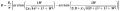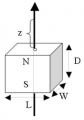# Calculation of the maximum detectable distance of a magnet by a magnetometer

#### siavosh1

Joined Apr 16, 2019
20
Hi Dear,
I have a single-component (Z-Axis) magnetometer with 60nT resolution.
I want to calculate the maximum distance that this magnetometer can detect an N52 magnet with a volume of 1 cm³.
So I used the following formula and inserted the values, and finally calculated the Z:Br = 1.47 T (for Magnet N52)
B= 60 nT (magnetometer resolution)
L=W=D=10mm
Z=?
My answer => Z= 1.579 m
Keep in mind that I intended to solve this problem in the simplest possible way; the angle of view of the magnetometer is perpendicular to the magnet plate.My question is whether I have chosen the right formula? or do you suggest another solution for it.